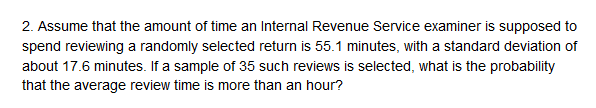that the amount of time an Internal Revenue Service examiner is supposed tospend reviewing a randomly selected return is 55.1 minutes, with a standard deviation ofabout 17.6 minutes. If a sample of 35 such reviews is selected, what is the probabilitythat the average review time is more than an hour?

Questionhelp_outlineImage Transcriptionclosethat the amount of time an Internal Revenue Service examiner is supposed to spend reviewing a randomly selected return is 55.1 minutes, with a standard deviation of about 17.6 minutes. If a sample of 35 such reviews is selected, what is the probability that the average review time is more than an hour? fullscreen
Step 1

Central Limit Theorem for mean:

If a random sample of size n is taken from a population having mean  and standard deviation  then, as the sample size increases, the sample mean approaches the normal distribution with mean  and standard deviation σ/ sqrt(n).

Step 2

Find the probability that the average review time is more than an hour:

The amount of time an internal revenue service examiner is supposed to spend reviewing a randomly selected return is 55.1 minutes with a standard deviation of 17.6 minutes. That is, mean μ = 55.1 minutes and the population standard deviation is σ = \$17.6 minutes.  Random samples of 35 (n) reviews are drawn from the population. Let the random variable X denotes the amount of time an internal revenue service examiner is supposed to spend reviewing a randomly selected return. By central limit theorem for mean, the mean time of the sample follows a normal distribution with mean μ = 55.1 and standard deviation 17.6/sqrt (35) = 2.97. Thus, the probability that the average review time is more than an hour (60 minutes) is calculated as follows:

Step 3

Thus, the probability that the average review...

Want to see the full answer?

See Solution

Want to see this answer and more?

Our solutions are written by experts, many with advanced degrees, and available 24/7

See Solution
Tagged in

Other